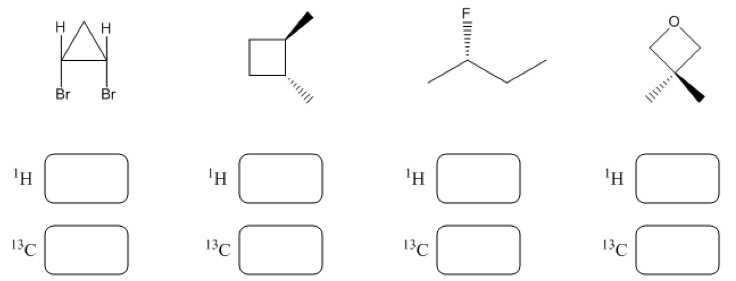# Problem: In each molecule below, identify the number of unique 1H and 13C NMR signals you would expect to observe on a spectrum and write the number in the box provided.

85% (17 ratings)
###### Problem Details

In each molecule below, identify the number of unique 1H and 13C NMR signals you would expect to observe on a spectrum and write the number in the box provided.What scientific concept do you need to know in order to solve this problem?

Our tutors have indicated that to solve this problem you will need to apply the 1H NMR: Number of Signals concept. You can view video lessons to learn 1H NMR: Number of Signals. Or if you need more 1H NMR: Number of Signals practice, you can also practice 1H NMR: Number of Signals practice problems.

What is the difficulty of this problem?

Our tutors rated the difficulty ofIn each molecule below, identify the number of unique 1H and...as medium difficulty.

How long does this problem take to solve?

Our expert Organic tutor, Jonathan took 8 minutes and 29 seconds to solve this problem. You can follow their steps in the video explanation above.

What professor is this problem relevant for?

Based on our data, we think this problem is relevant for Professor Mascall's class at Brandeis University.